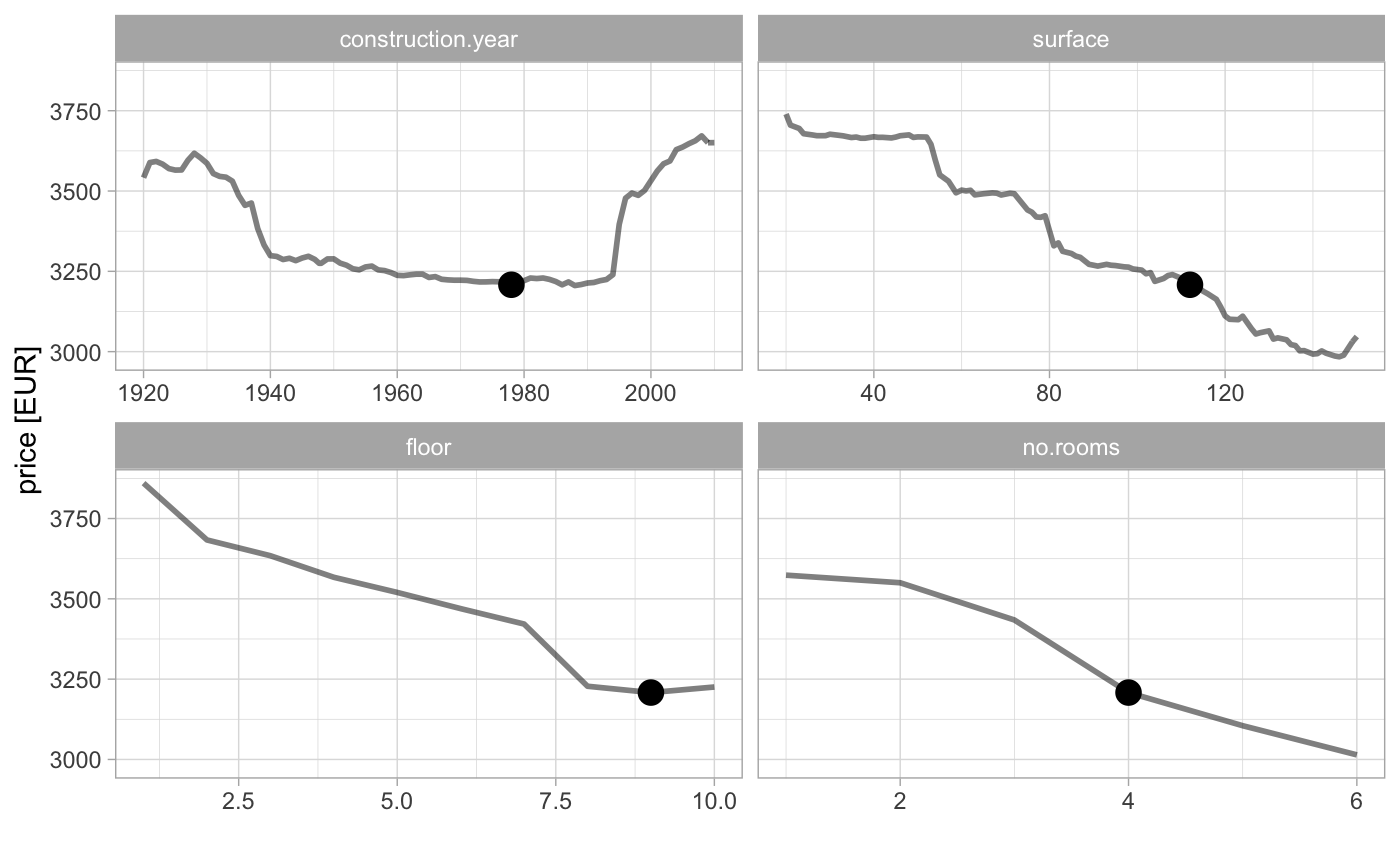# Introduction

First, let’s create explainers for random Forest model and linear model

library("DALEX")
library("randomForest")
library("ceterisParibus")
set.seed(59)

# set default theme
theme_set(theme_bw() + theme(text = element_text(size = 18)))

# preprare model
apartments_rf_model <- randomForest(m2.price ~ construction.year + surface + floor +
no.rooms + district,
data = apartments)

explainer_rf <- explain(apartments_rf_model,
data = apartmentsTest[,2:6],
y = apartmentsTest$m2.price) # Simple plots Let’s start with plots for a single observation. apartments_A <- apartmentsTest[958,] cp_rf_A <- ceteris_paribus(explainer_rf, apartments_A, y = apartments_A$m2.price)

plot(cp_rf_A, show_profiles = TRUE, show_observations = TRUE,
selected_variables = c("surface","construction.year"))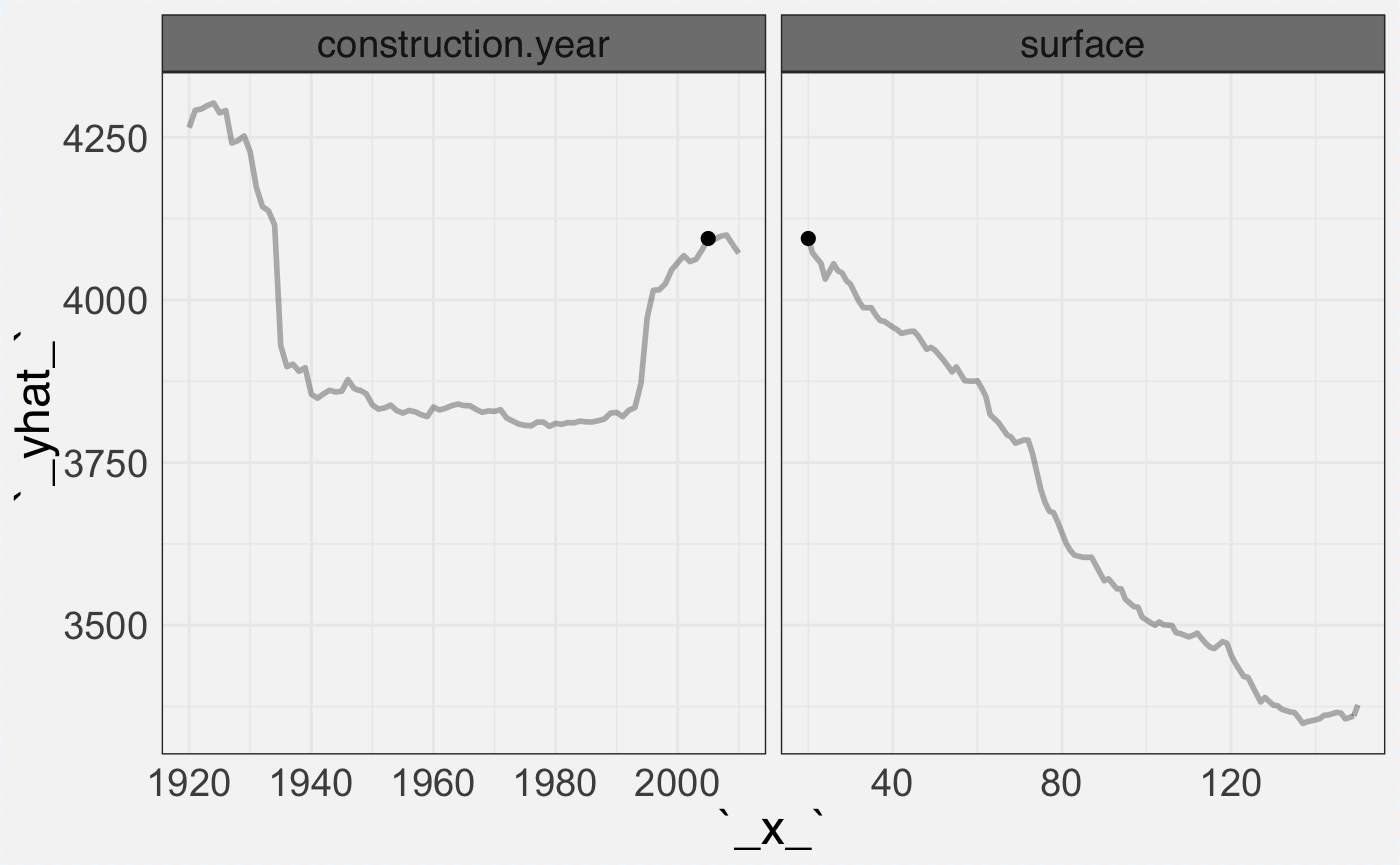Here are plots for neighbours of this observation.

apartments_B <- select_neighbours(apartmentsTest, apartmentsTest[958,], n = 15)
cp_rf_B <- ceteris_paribus(explainer_rf, apartments_B, y = apartments_B$m2.price) plot(cp_rf_B, show_profiles = TRUE, show_observations = TRUE, show_rugs = TRUE, selected_variables = c("surface","construction.year"))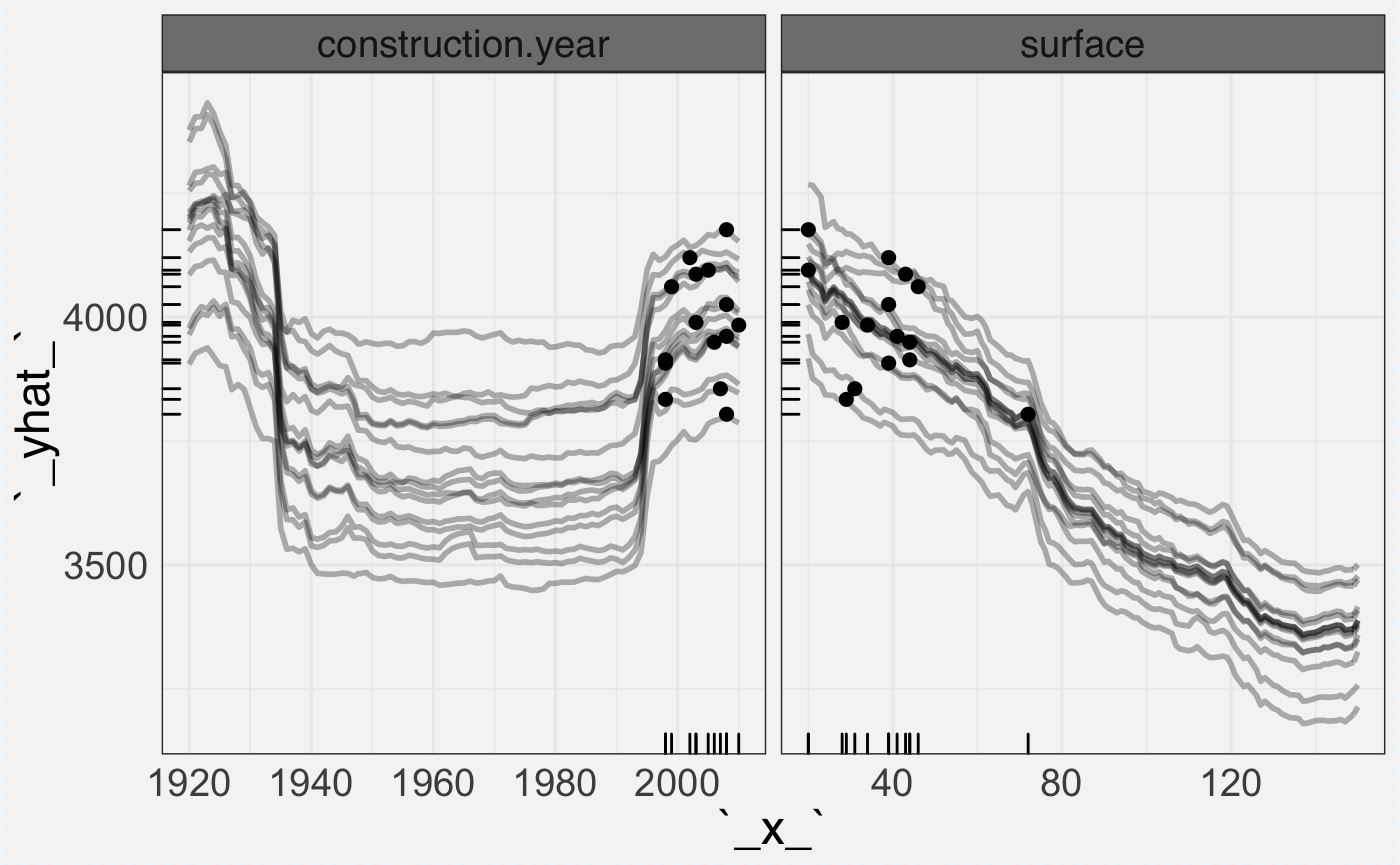Here we have an example for a subset of observations and only points. apartments_C <- select_sample(apartmentsTest, n = 15) cp_rf_C <- ceteris_paribus(explainer_rf, apartments_C, y = apartments_C$m2.price)

plot(cp_rf_C,
show_profiles = FALSE,
selected_variables = c("surface","construction.year"))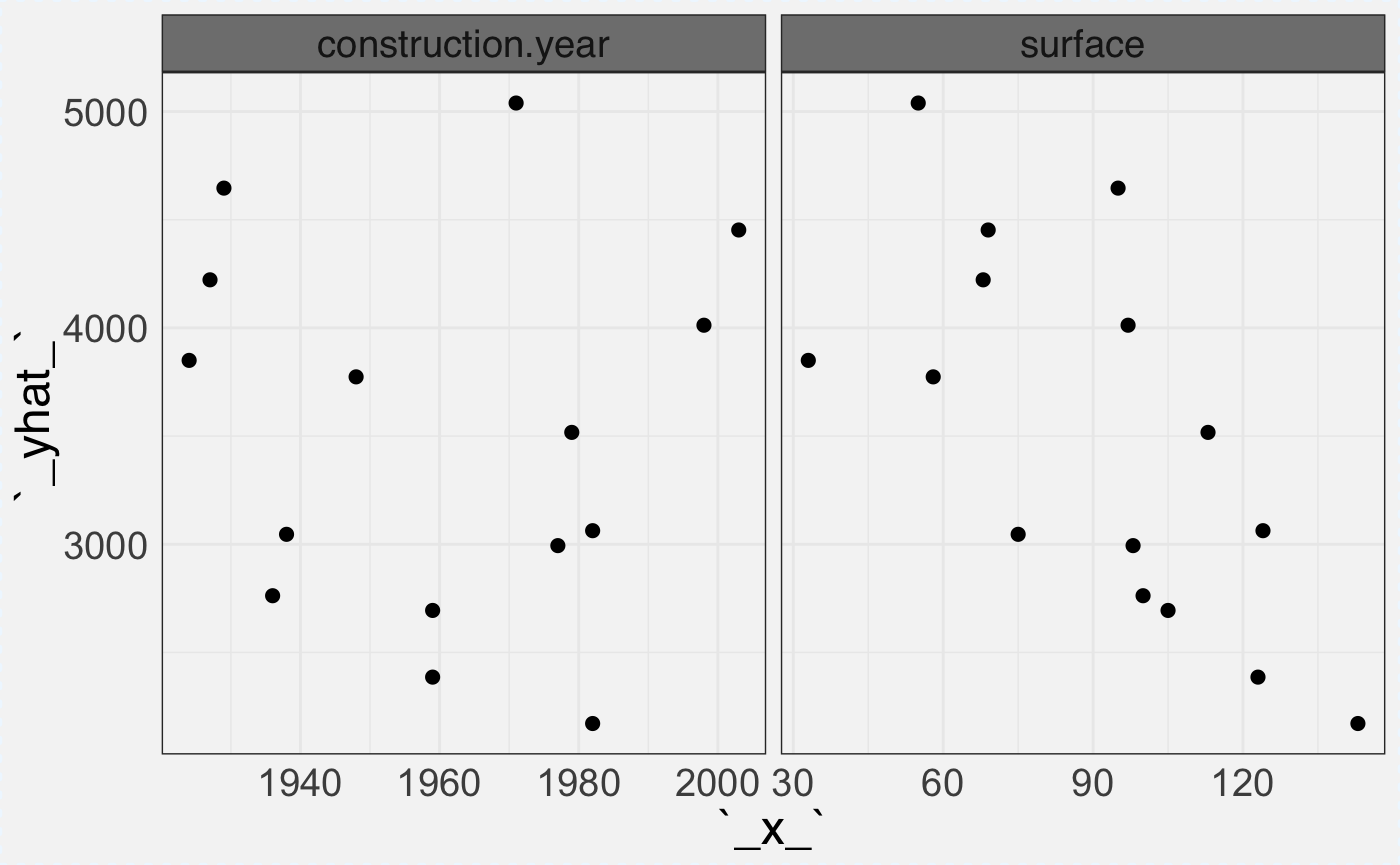Only rugs

plot(cp_rf_C,
show_profiles = FALSE, show_observations = FALSE, show_rugs = TRUE,
selected_variables = c("surface","construction.year"))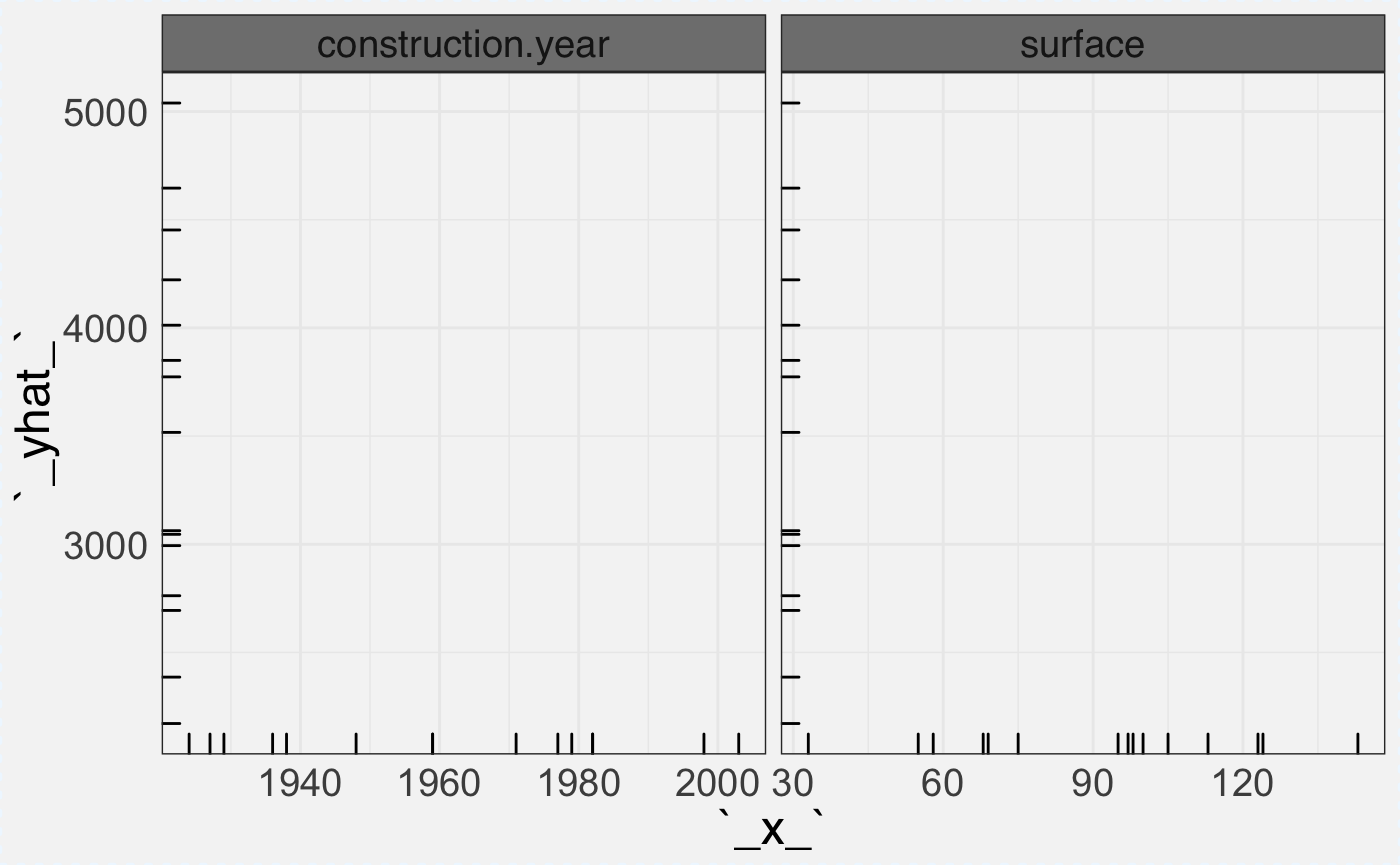Only profiles

plot(cp_rf_C,
show_profiles = TRUE, show_observations = FALSE,
selected_variables = c("surface","construction.year"))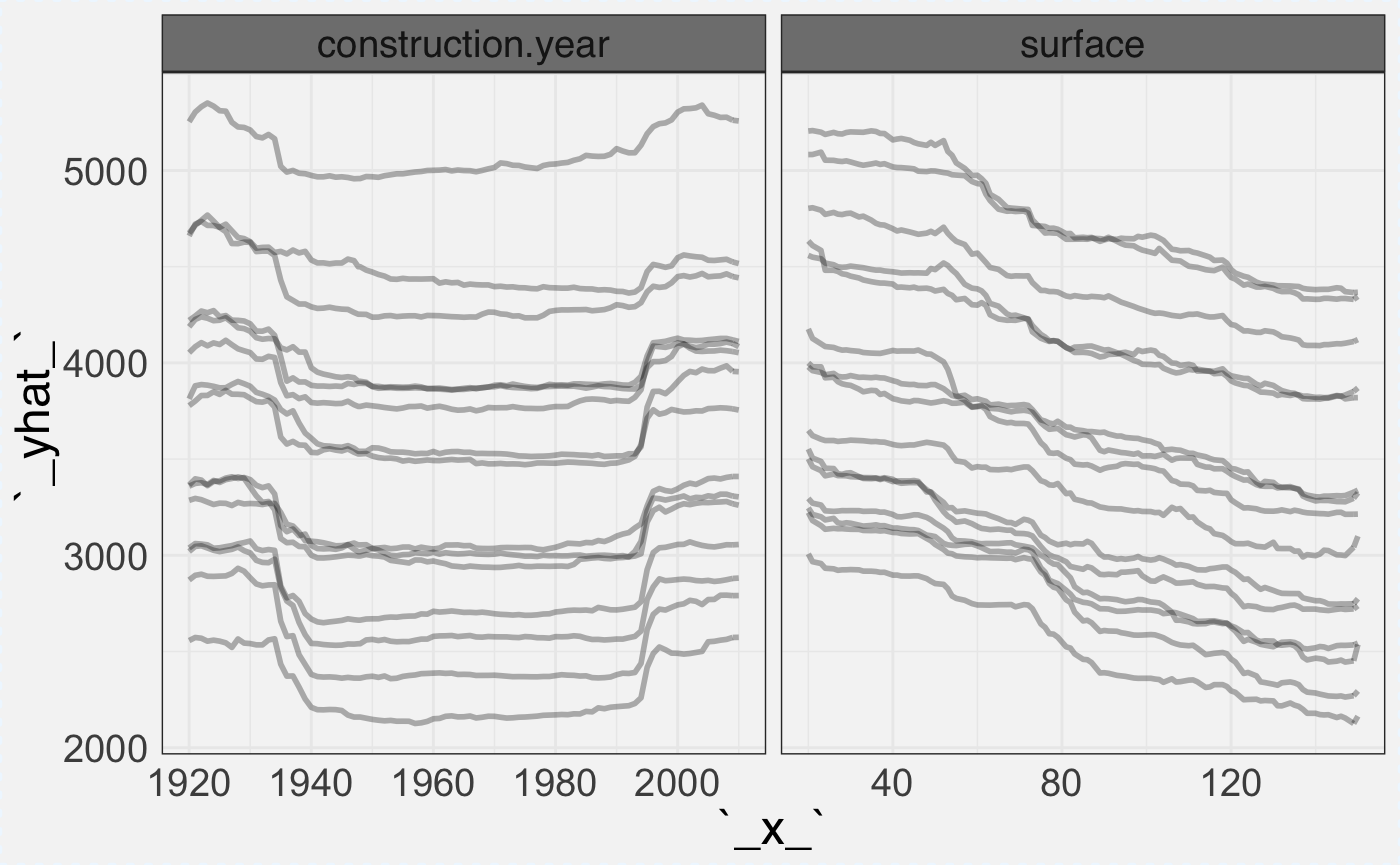Only residuals

plot(cp_rf_C,
show_profiles = FALSE, show_observations = FALSE, show_residuals = TRUE,
selected_variables = c("surface","construction.year"))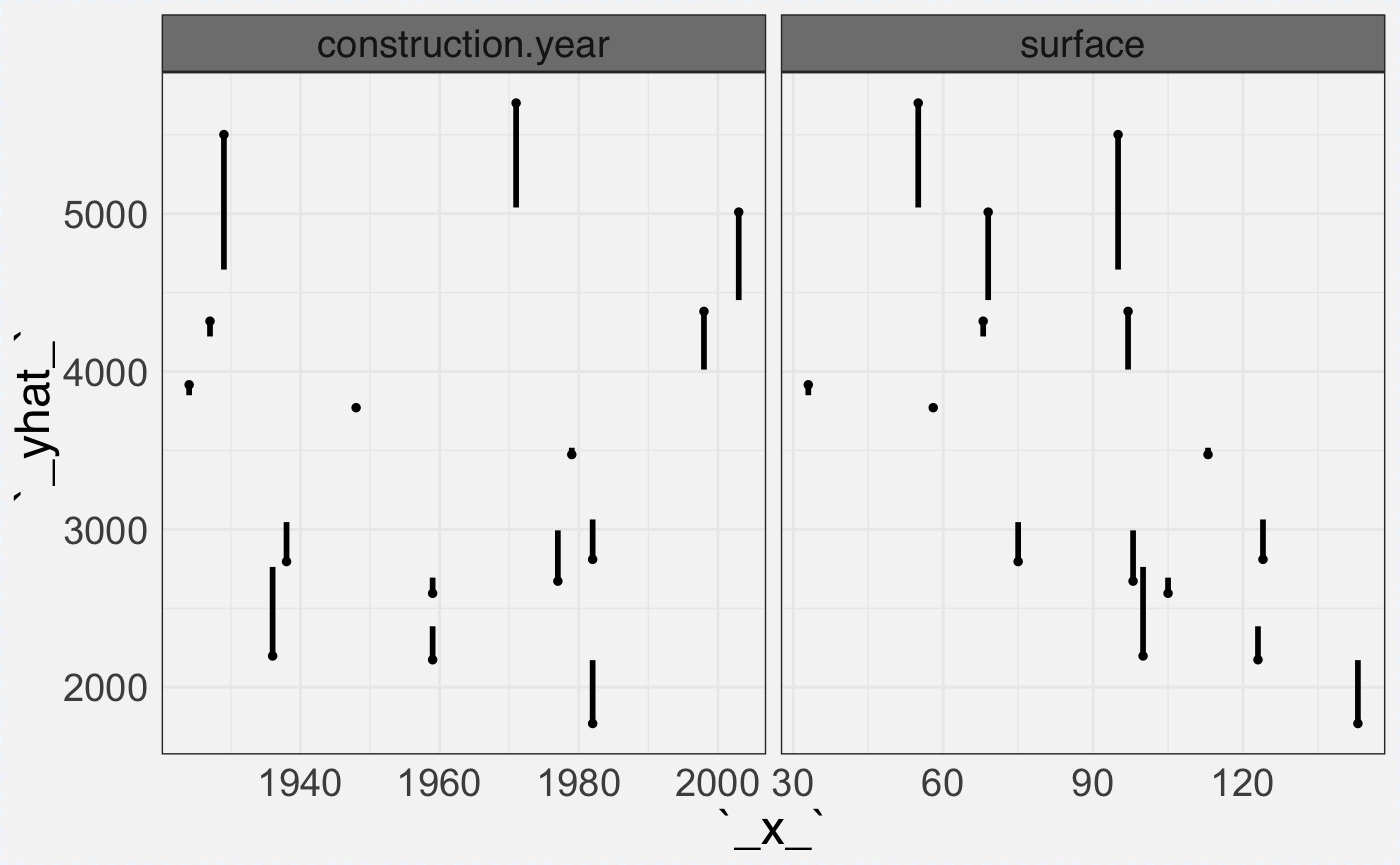Only average profile

plot(cp_rf_C,
show_profiles = TRUE, show_observations = FALSE,
aggregate_profiles = mean, size = 2,
selected_variables = c("surface","construction.year"))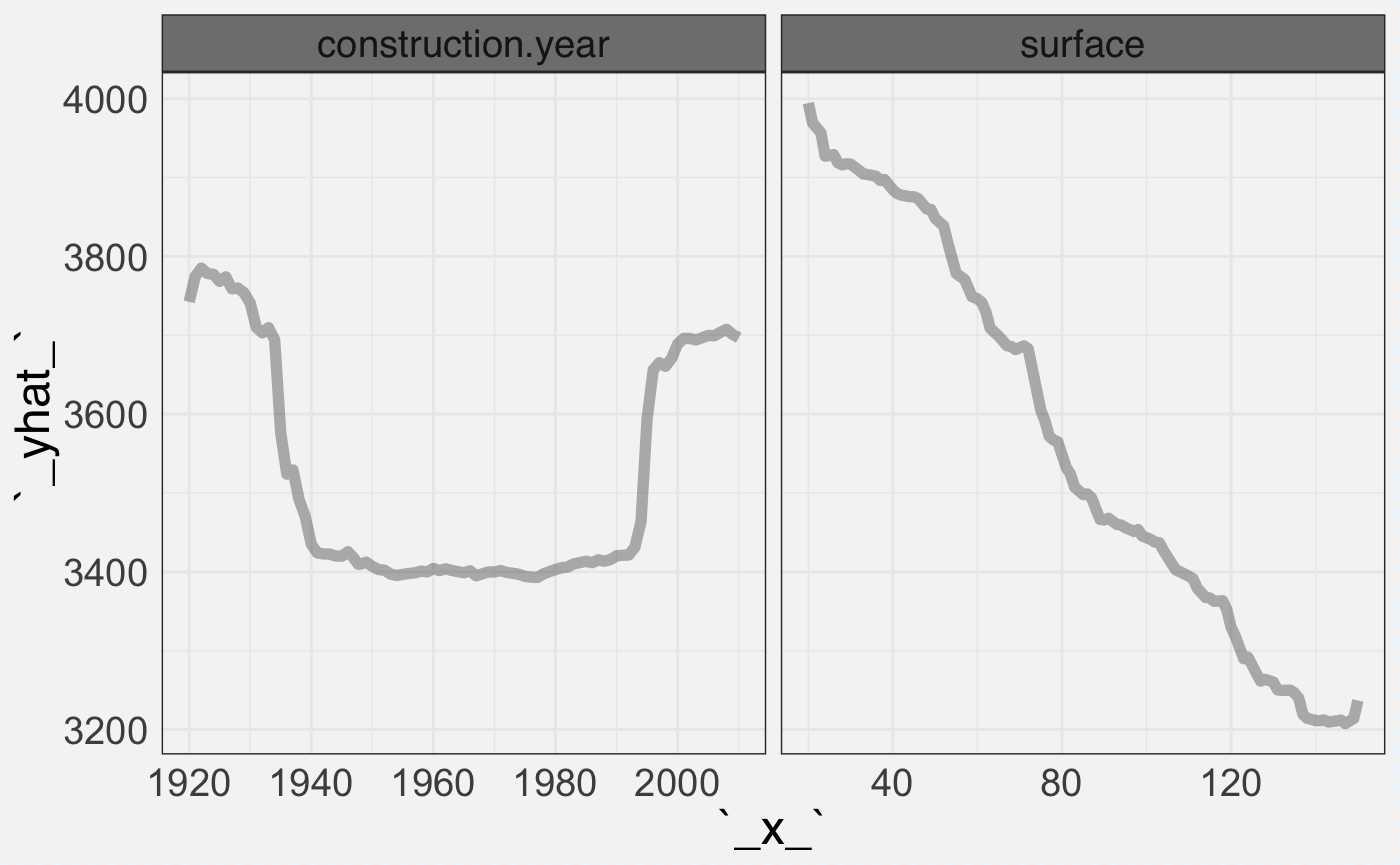Colors correspond to district

plot(cp_rf_C,
show_profiles = TRUE, show_observations = FALSE,
color = "district", alpha = 1,
selected_variables = c("surface","construction.year", "district"))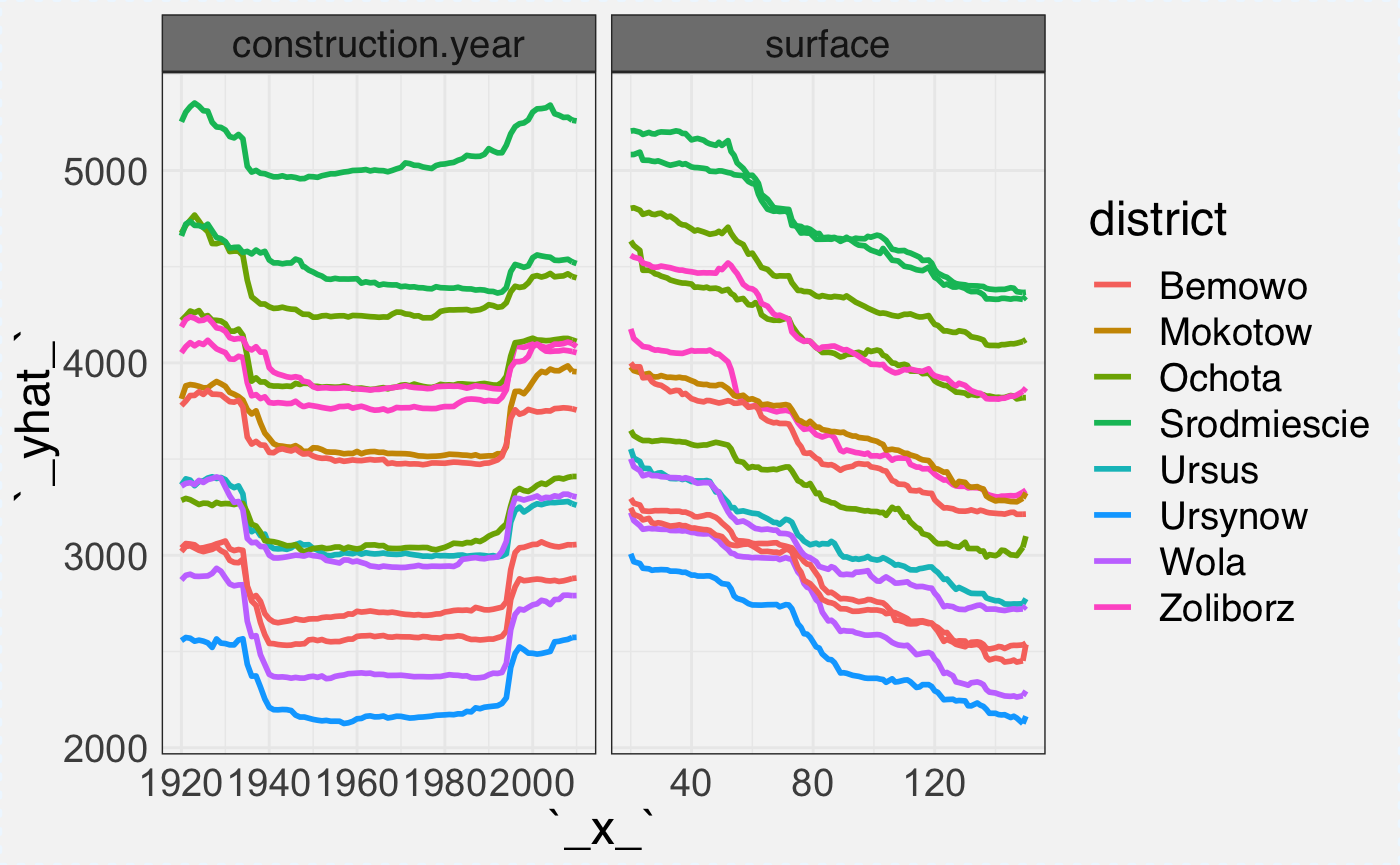Colors correspond to surface.

plot(cp_rf_C,
show_profiles = TRUE, show_observations = TRUE,
color = "surface", alpha = 1,
selected_variables = c("surface","construction.year"))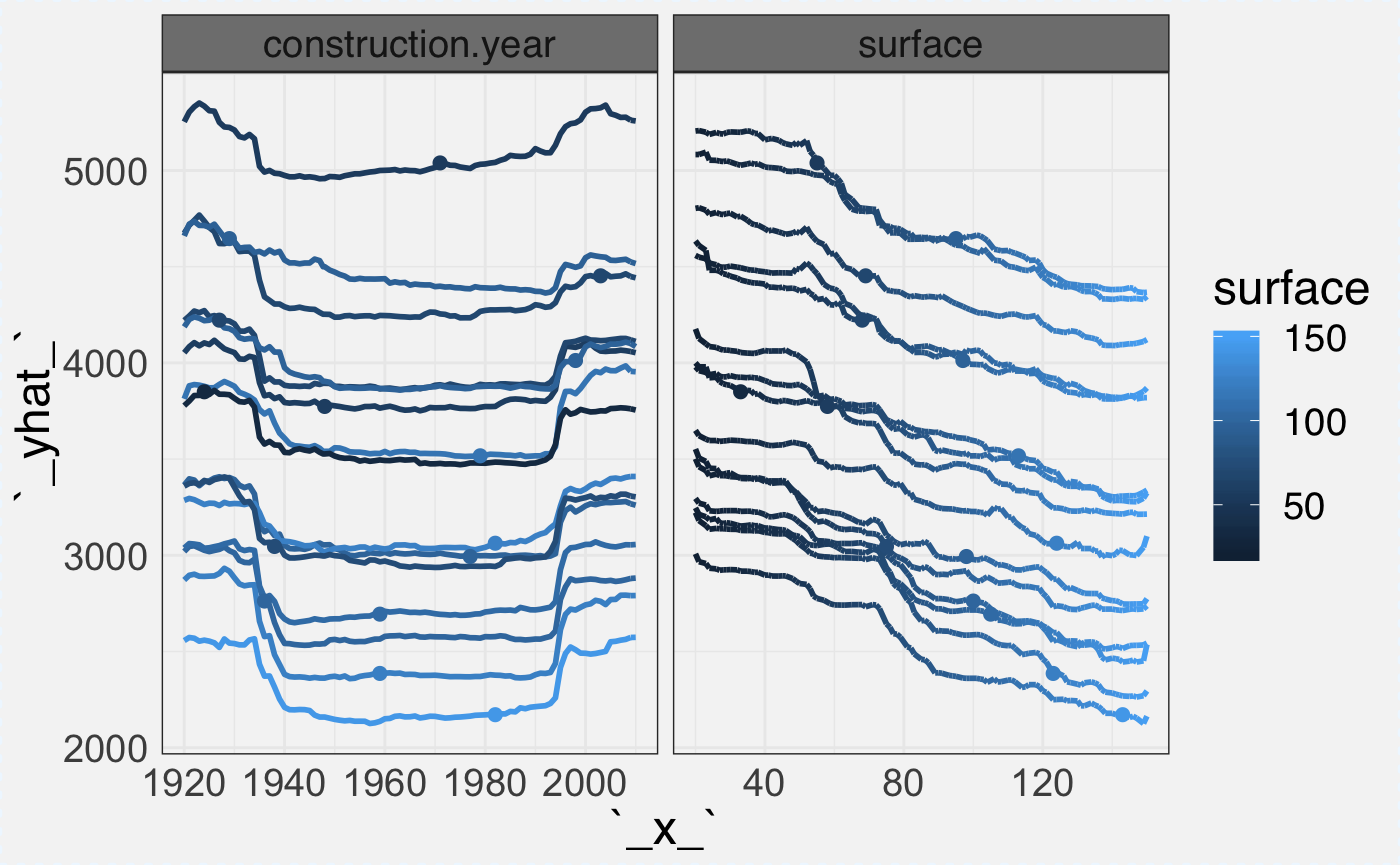Observations without rugs.

plot(cp_rf_C,
show_profiles = TRUE, show_observations = TRUE,
selected_variables = c("surface","construction.year"))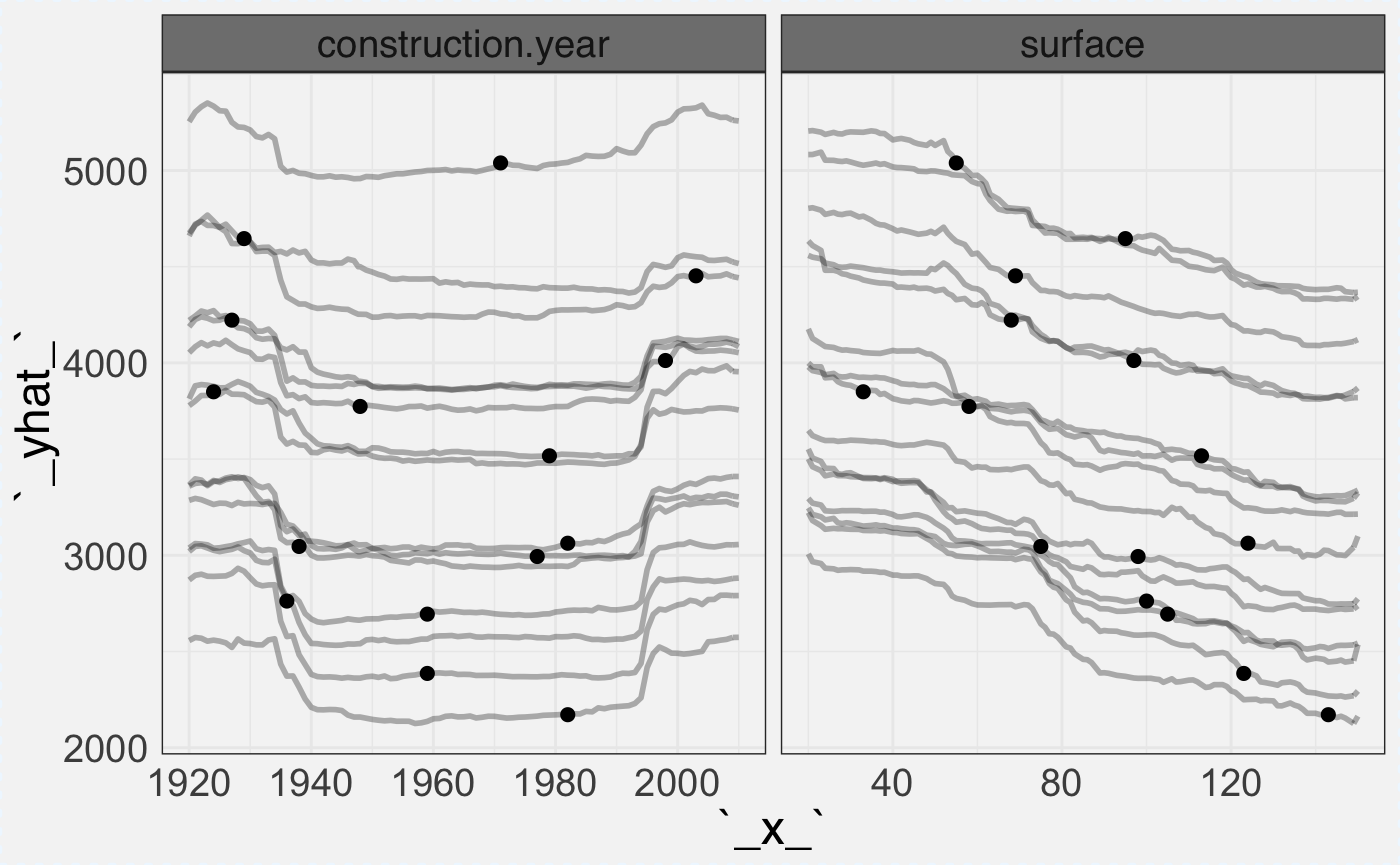Different colors for different elements.

plot(cp_rf_C,
show_profiles = TRUE, show_observations = TRUE, show_rugs = TRUE,
show_residuals = TRUE,
color = "blue", color_points = "orange", color_residuals = "red", color_rugs = "green",
alpha = 0.3, alpha_points = 0.3, alpha_residuals = 0.5, alpha_rugs = 1,
size_points = 4, size_rugs = 0.5,
selected_variables = c("surface","construction.year"))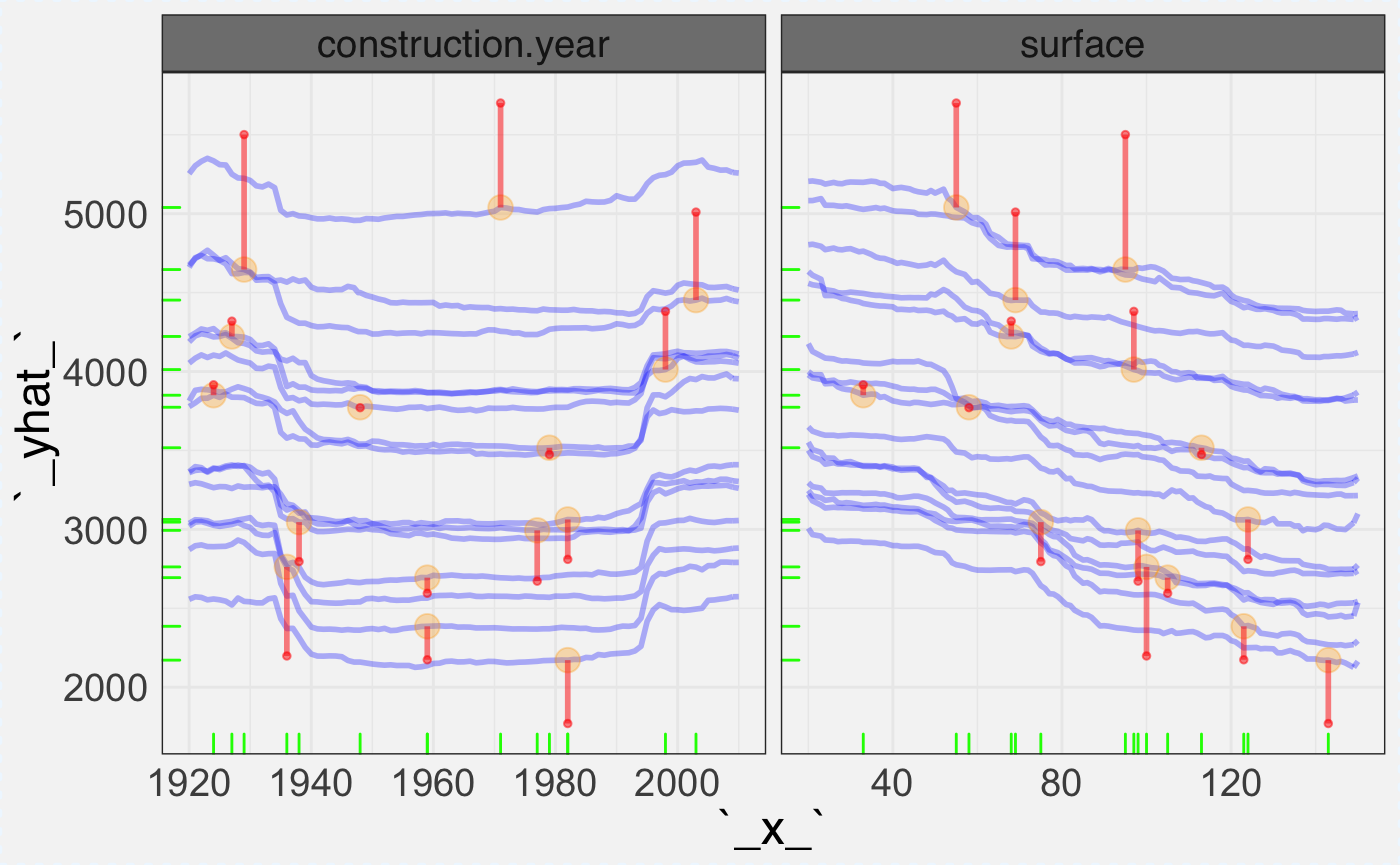# Complex plots

Here we are going to mix two or more layers.

First one presents individual profiles and the average between profiles.

# mixtures

plot(cp_rf_C,
show_observations = FALSE, show_rugs = TRUE,
show_residuals = TRUE, color_residuals = "red", size_residuals = 2,
selected_variables = c("surface","construction.year")) +
ceteris_paribus_layer(cp_rf_C,
show_observations = FALSE, show_rugs = FALSE,
aggregate_profiles = mean, size = 2, alpha = 1,
selected_variables = c("surface","construction.year"))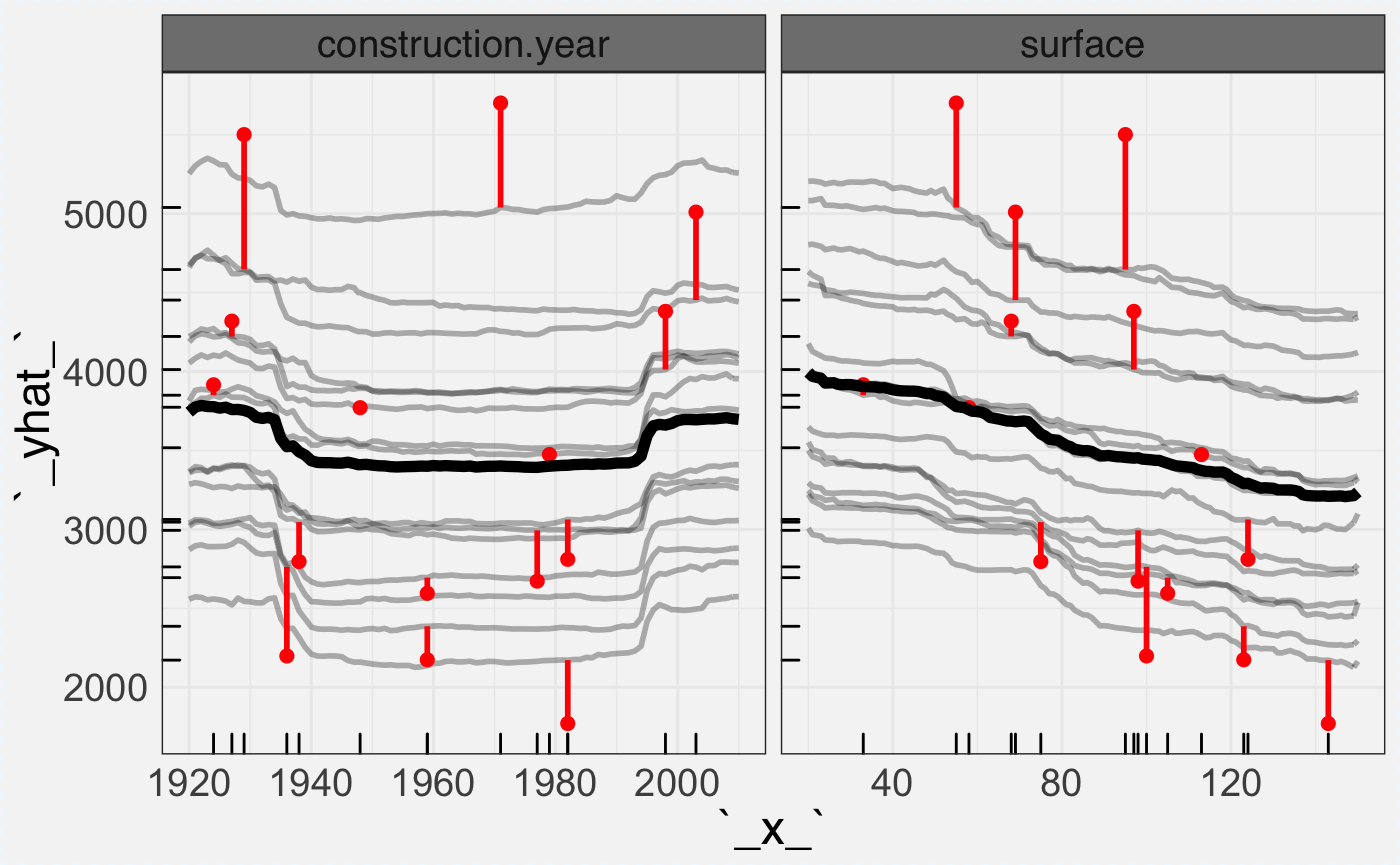Neighbourings for two observations.

apartments_D <- select_neighbours(apartmentsTest, apartmentsTest[348,], n = 15)
cp_rf_D <- ceteris_paribus(explainer_rf, apartments_D, y = apartments_D$m2.price) plot(cp_rf_B, show_observations = FALSE, show_residuals = TRUE, color_residuals = "blue", selected_variables = c("surface","construction.year")) + ceteris_paribus_layer(cp_rf_D, show_observations = FALSE, show_residuals = TRUE, color = "orange", color_residuals = "red", selected_variables = c("surface","construction.year"))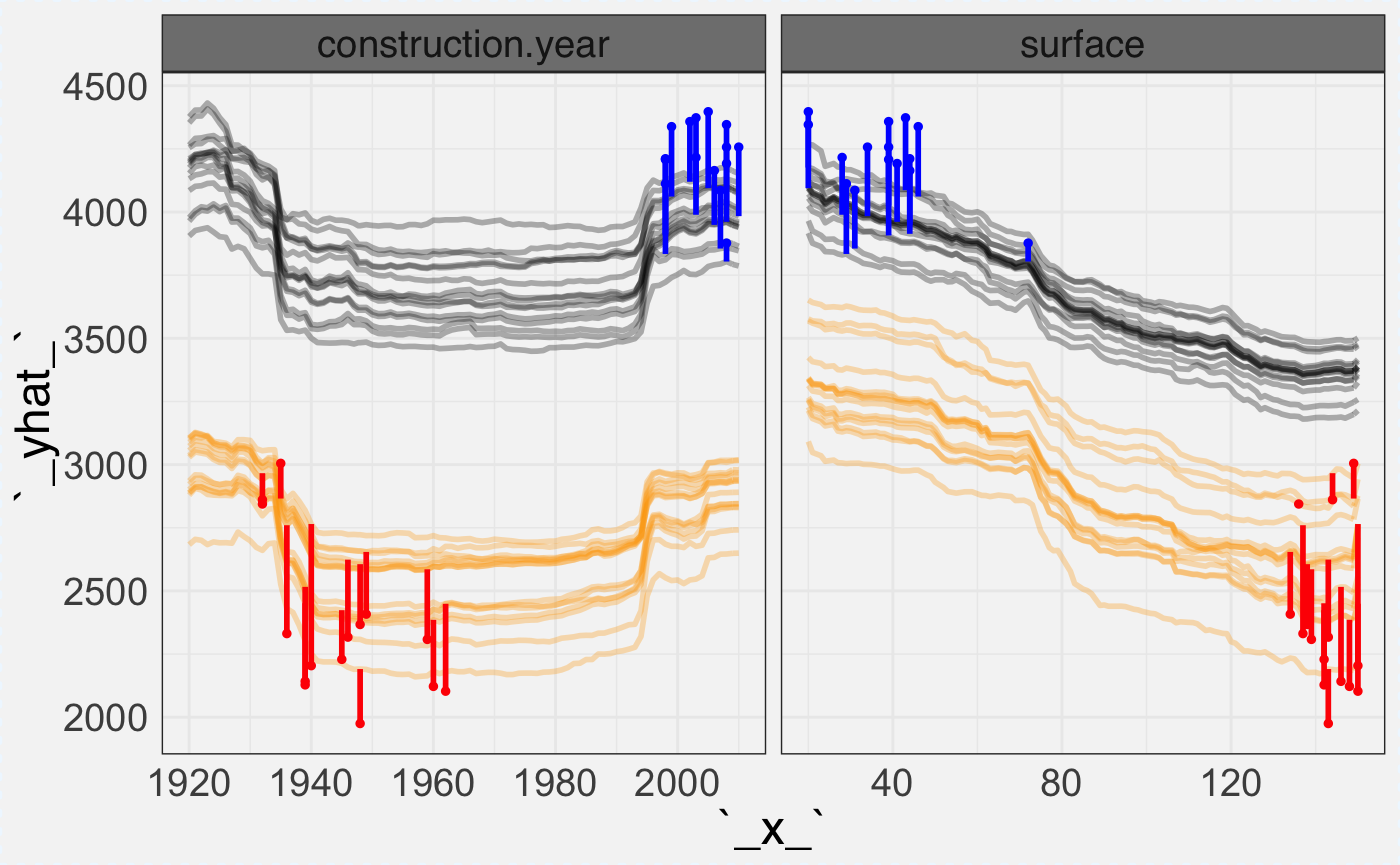Profiles for two models: random forest and SVM. library("rpart") library("e1071") apartments_svm_model <- svm(m2.price ~ construction.year + surface + floor + no.rooms + district, data = apartments) apartments_rpart_model <- best.rpart(m2.price ~ construction.year + surface + floor + no.rooms + district, data = apartments) explainer_svm <- explain(apartments_svm_model, data = apartmentsTest[,2:6], y = apartmentsTest$m2.price)

explainer_rpart <- explain(apartments_rpart_model,
data = apartmentsTest[,2:6], y = apartmentsTest$m2.price) apartments_E <- apartmentsTest[958,] cp_rf_E <- ceteris_paribus(explainer_svm, apartments_E, y = apartments_E$m2.price)

apartments_F <- apartmentsTest[958,]
cp_rpart_F <- ceteris_paribus(explainer_rpart, apartments_F, y = apartments_F$m2.price) plot(cp_rf_A, show_observations = FALSE, show_rugs = TRUE, show_residuals = TRUE, color_residuals = "blue", selected_variables = c("surface","construction.year")) + ceteris_paribus_layer(cp_rf_E, show_observations = FALSE, show_rugs = TRUE, show_residuals = TRUE, color = "orange", color_residuals = "red", selected_variables = c("surface","construction.year"))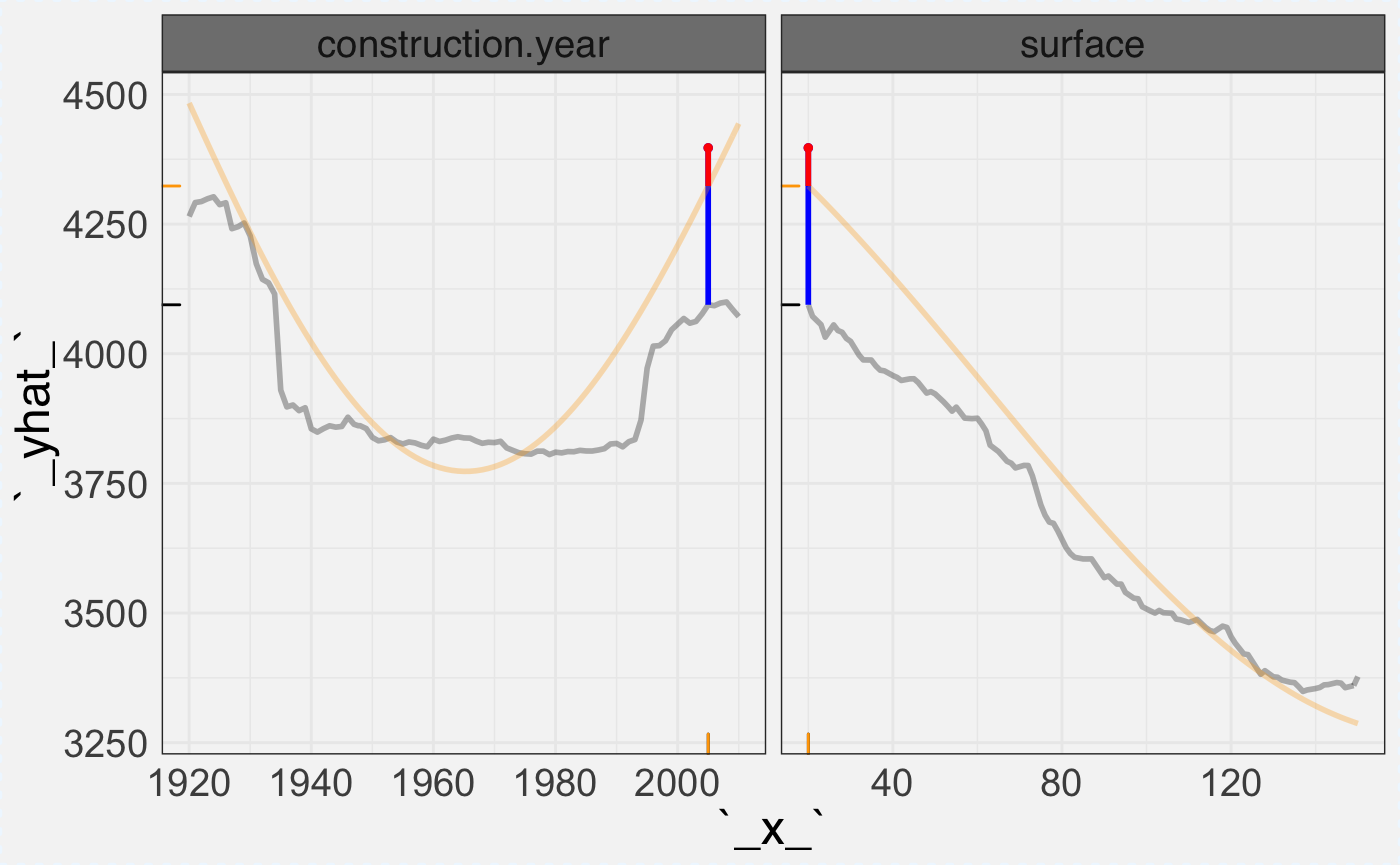Both models in a single layer plot(cp_rf_A, cp_rf_E, cp_rpart_F, color = "_label_", selected_variables = c("surface","construction.year"))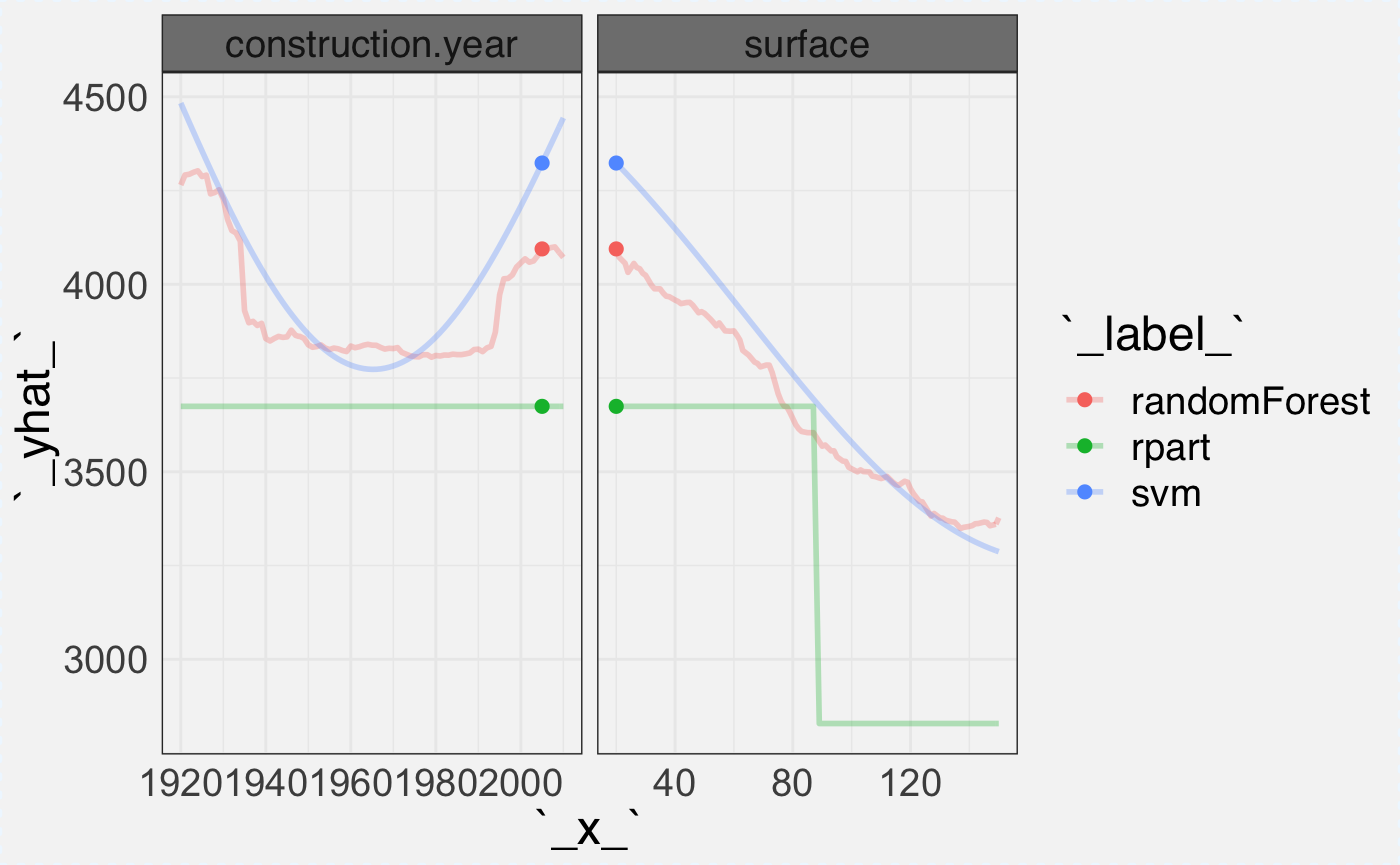# Cheatsheet Images and codes used in the cheatsheet apartments_A <- apartmentsTest[2,] cp_rf_A <- ceteris_paribus(explainer_rf, apartments_A, y = apartments_A$m2.price)

plot(cp_rf_A,
alpha = 0.5, size_points = 4,
selected_variables = "surface",
as.gg = TRUE) + xlab("") + ylab("price [EUR]") + theme_light()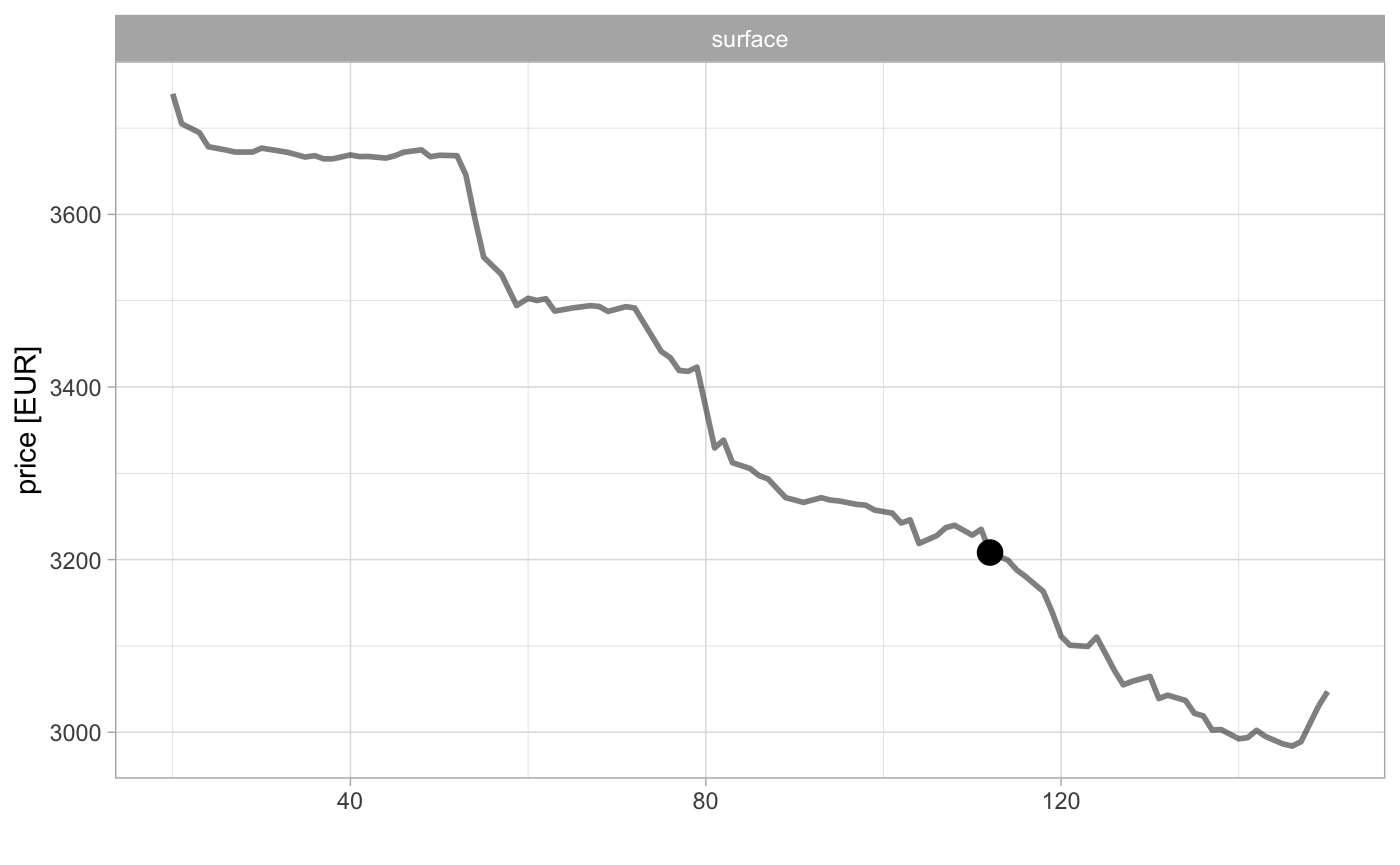apartments_B <- select_neighbours(apartmentsTest, apartments[1,], n = 10)
cp_rf_B <- ceteris_paribus(explainer_rf, apartments_B, y = apartments_B$m2.price) plot(cp_rf_B, show_rugs = TRUE, show_residuals = TRUE, selected_variables = "surface", color_residuals = "red", size = 0.5, as.gg = TRUE) + xlab("") + ylab("price [EUR]") + theme_light()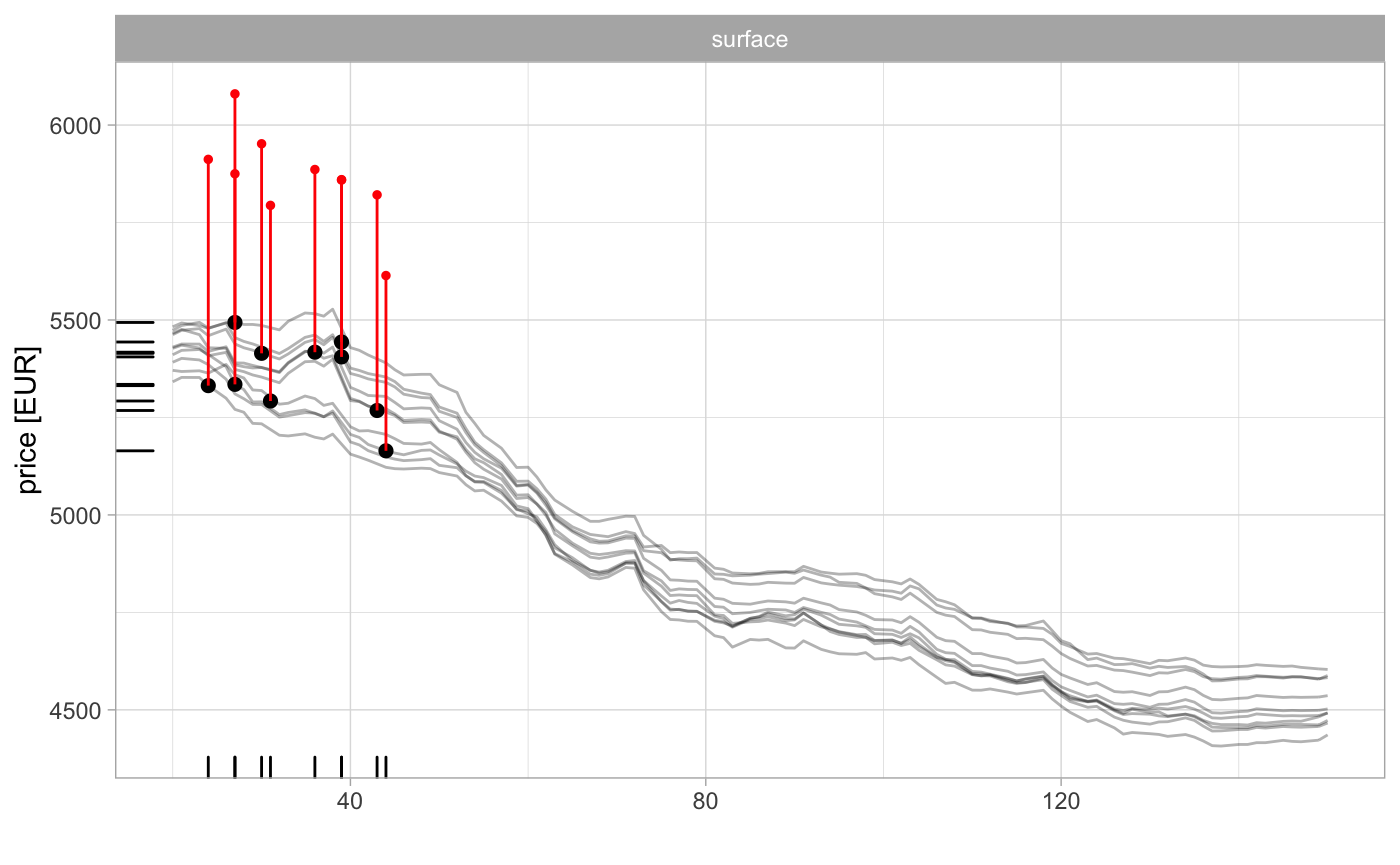apartments_C <- select_sample(apartmentsTest, n = 15) cp_rf_C <- ceteris_paribus(explainer_rf, apartments_C, y = apartments_C$m2.price)

plot(cp_rf_C,
show_observations = TRUE, show_rugs = TRUE, size = 0.3, size_points = 0.5,
selected_variables = "surface") +
ceteris_paribus_layer(cp_rf_C,
show_observations = FALSE,
aggregate_profiles = mean, size = 2, alpha = 1,
selected_variables = "surface",
as.gg = TRUE) + xlab("") + ylab("price [EUR]") + theme_light()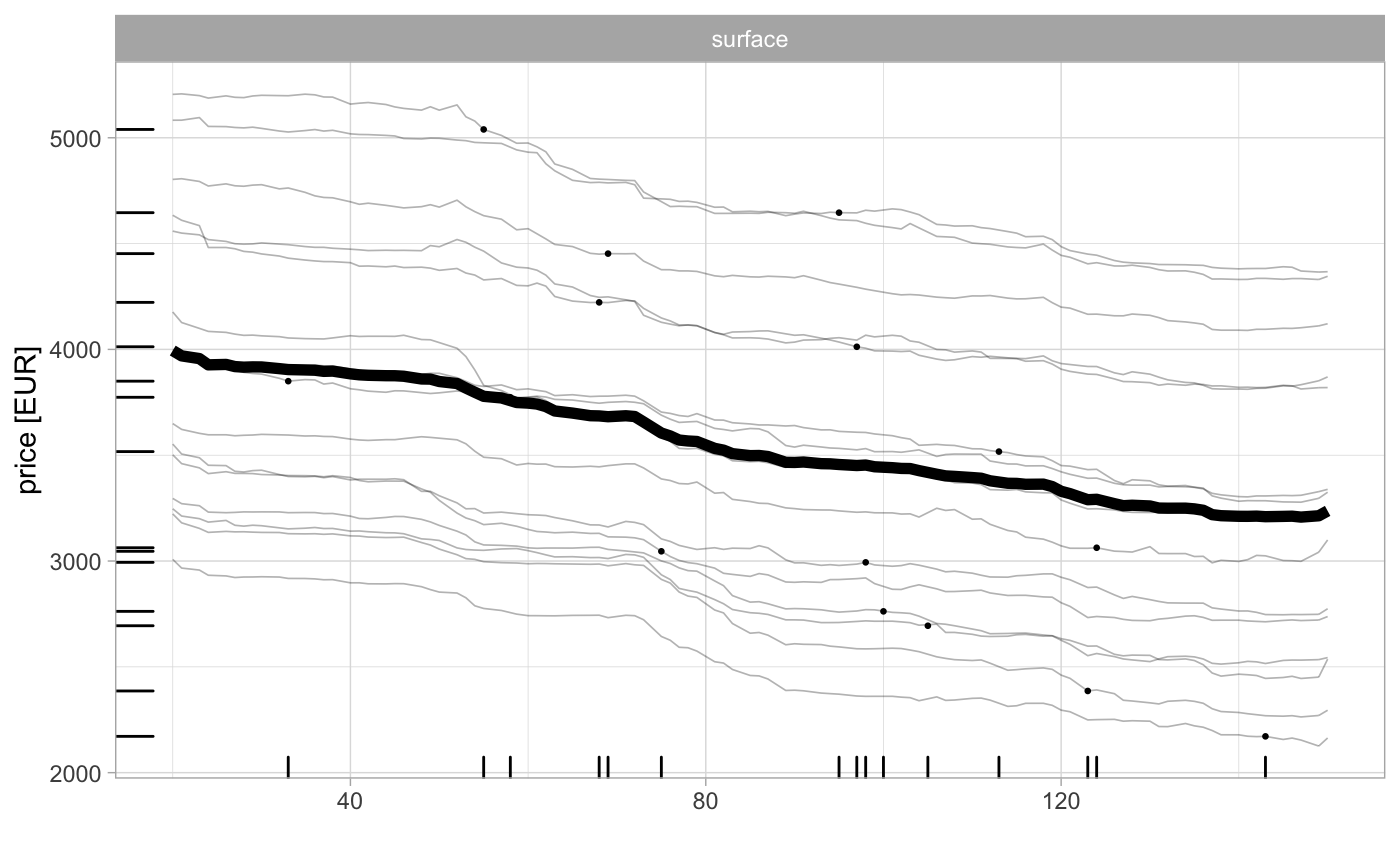apartments_D <- select_neighbours(apartmentsTest, apartmentsTest[348,], n = 15)
cp_rf_D <- ceteris_paribus(explainer_rf, apartments_D, y = apartments_D$m2.price) plot(cp_rf_B, show_observations = FALSE, show_residuals = TRUE, color_residuals = "blue", alpha = 0.5, size = 0.5, selected_variables = "surface") + ceteris_paribus_layer(cp_rf_D, show_observations = FALSE, show_residuals = TRUE, color = "orange", color_residuals = "red", alpha = 0.5, size = 0.5, selected_variables = "surface", as.gg = TRUE) + xlab("") + theme_light() + ylab("price [EUR]")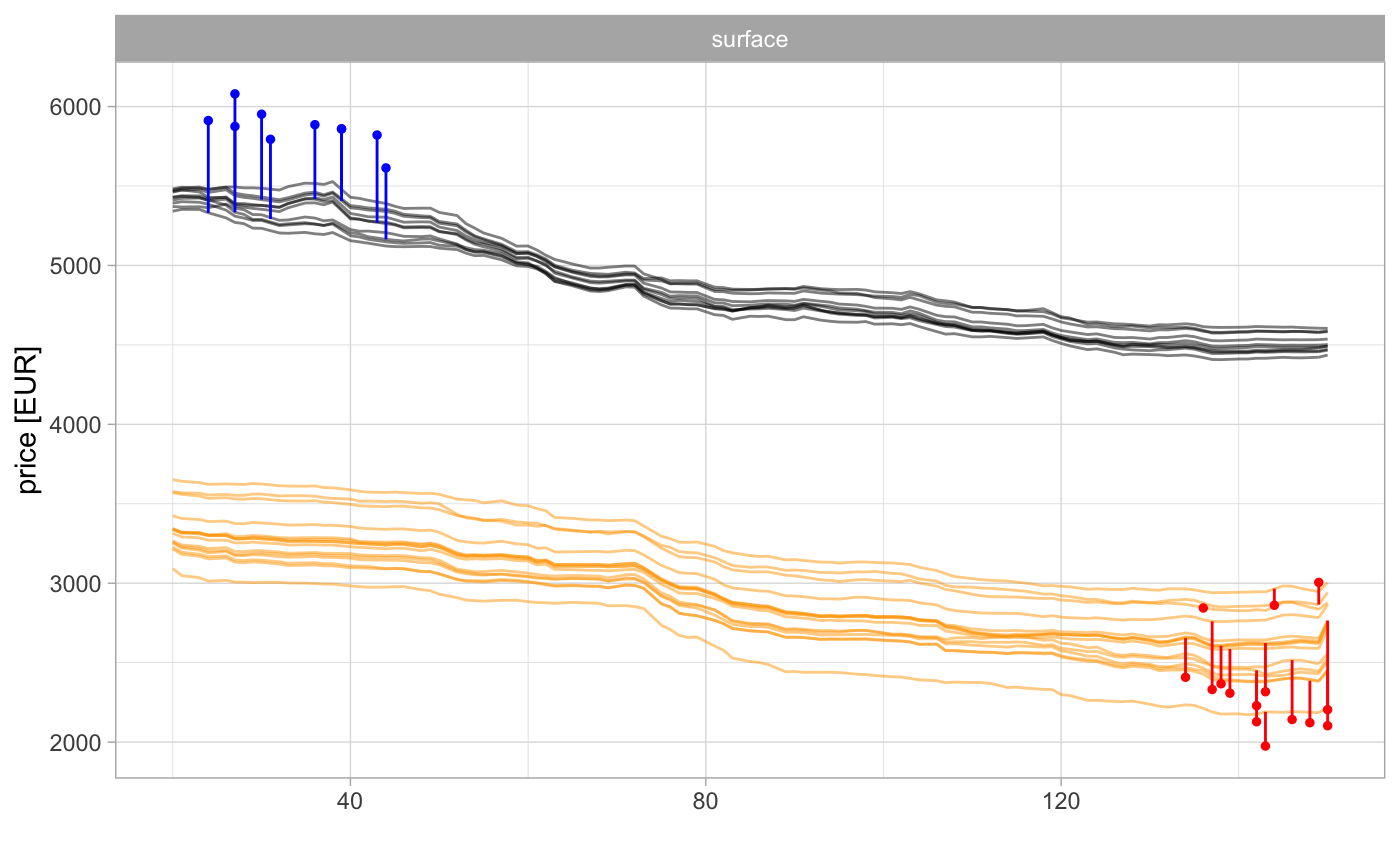plot(cp_rf_A, cp_rf_E, cp_rpart_F, color = "_label_", alpha = 0.5, size_points = 4, selected_variables = "surface", as.gg = TRUE) + xlab("") + ylab("price [EUR]") + theme_light() + scale_color_discrete(name="")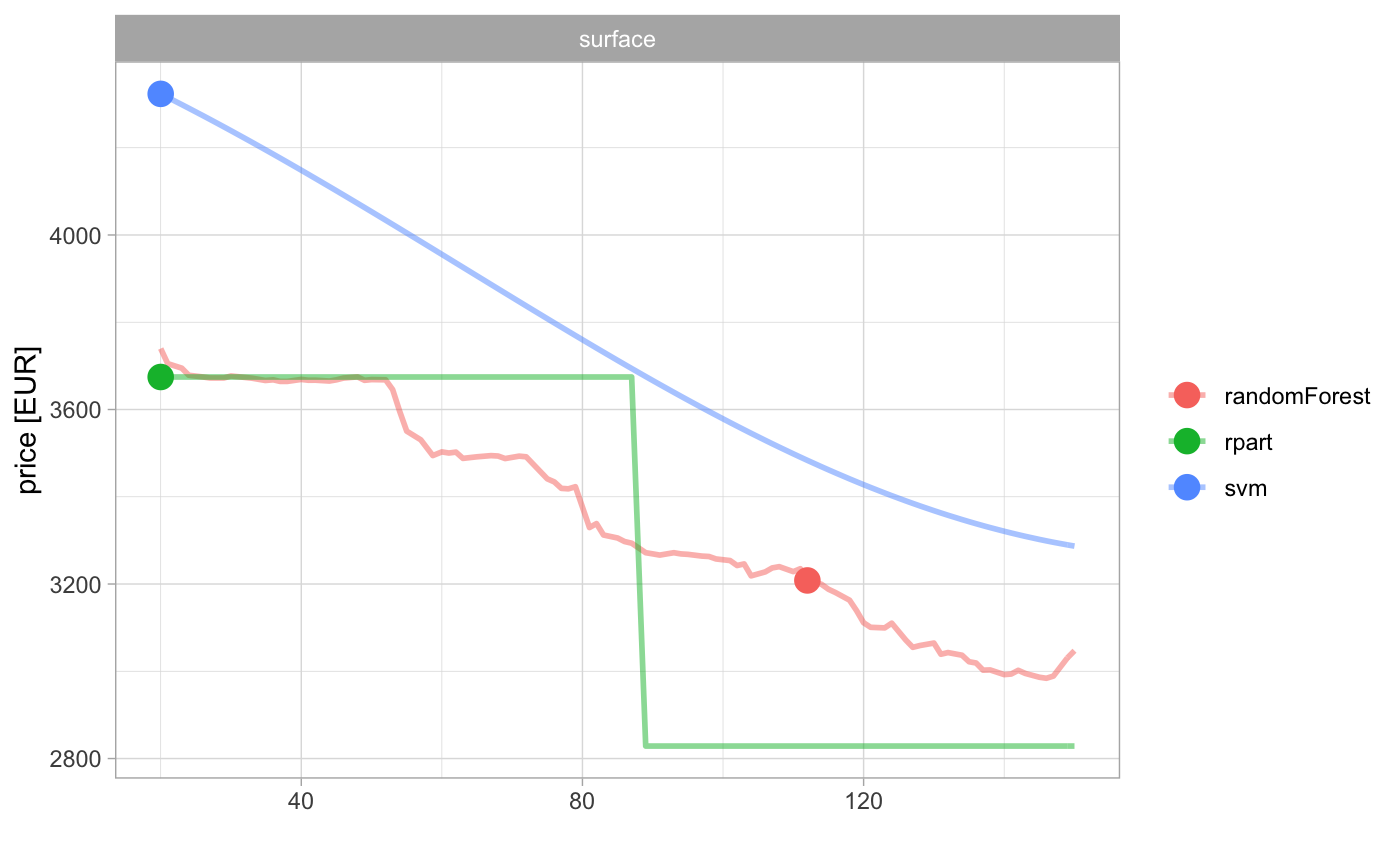plot(cp_rf_C[as.numeric(cp_rf_C$district) < 8,],
show_profiles = TRUE, show_observations = FALSE,
color = "district", alpha = 1,
selected_variables = c("surface", "district"),
as.gg = TRUE) + xlab("") + ylab("price [EUR]") + theme_light()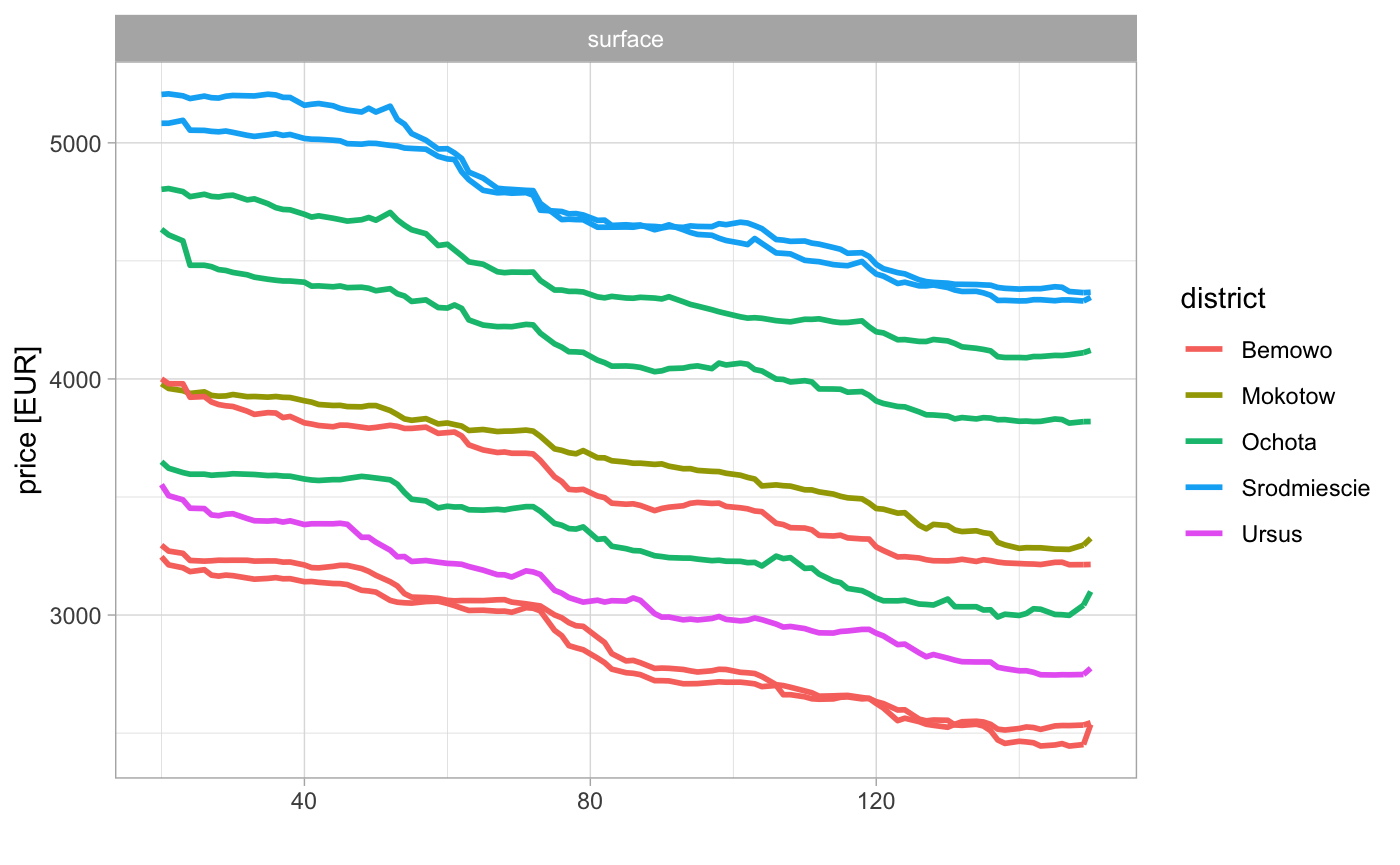plot(cp_rf_A,
alpha = 0.5, size_points = 4,
as.gg = TRUE) + xlab("") + ylab("price [EUR]") + theme_light()